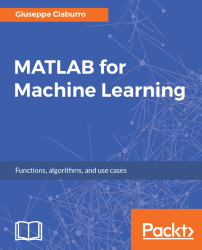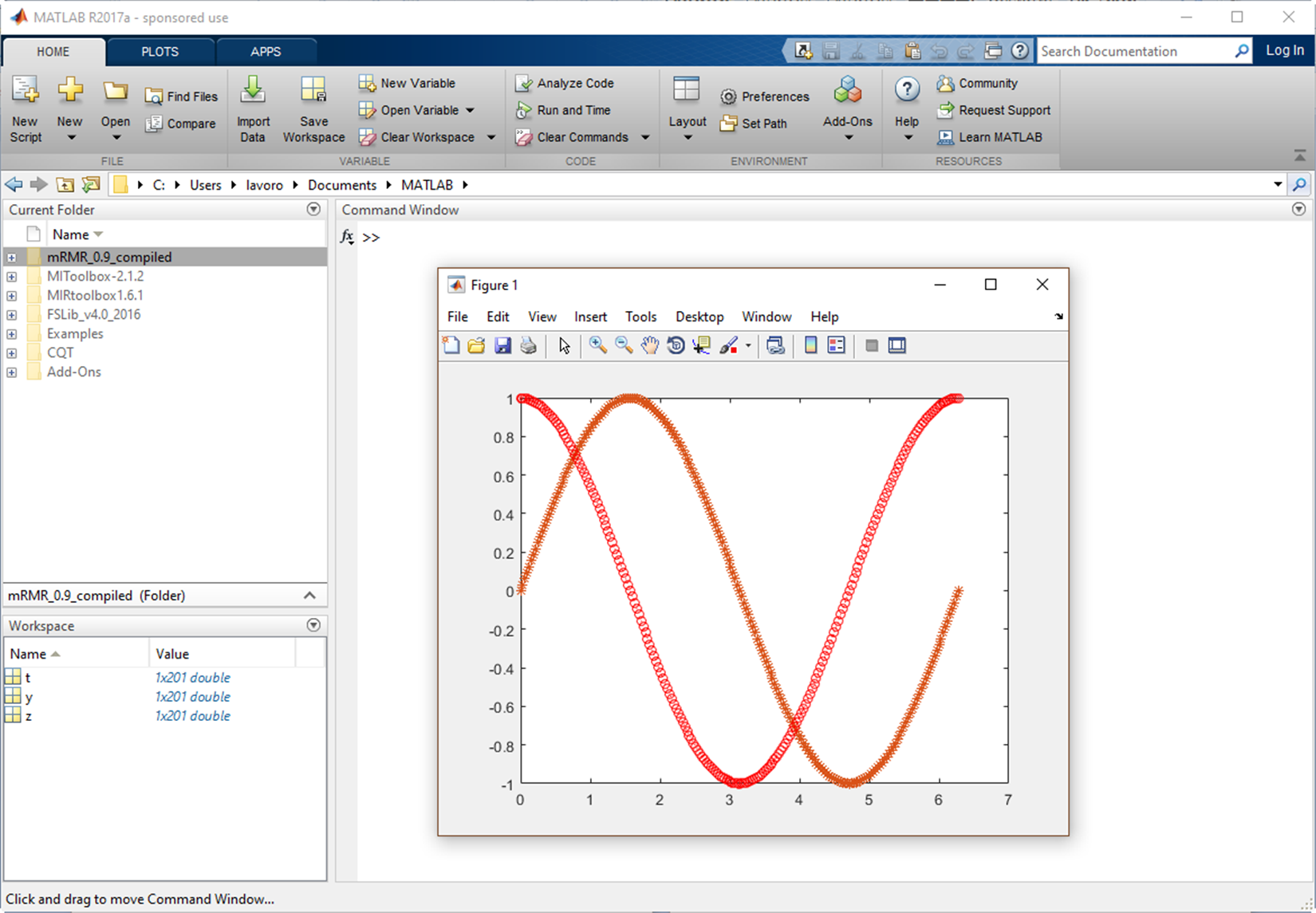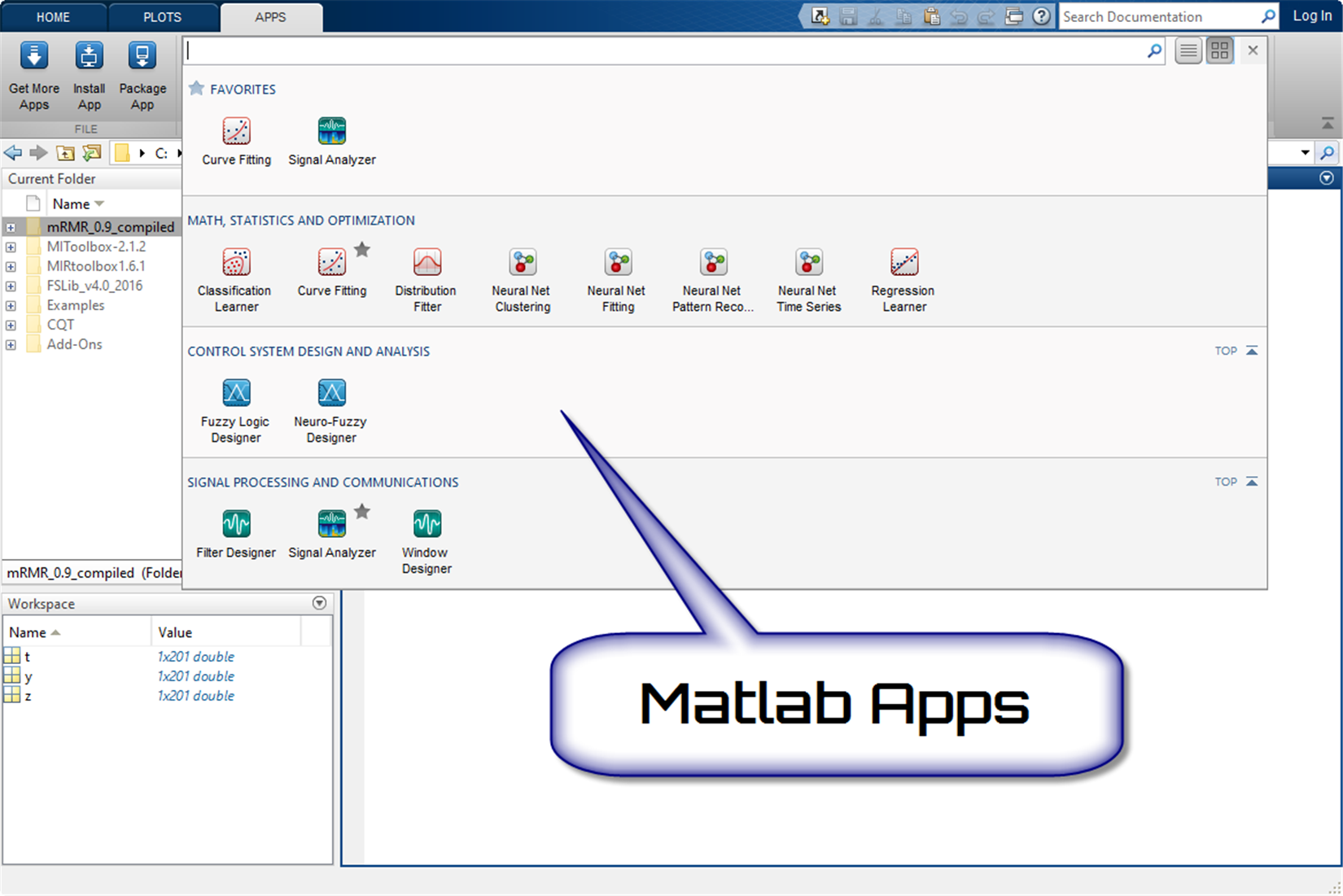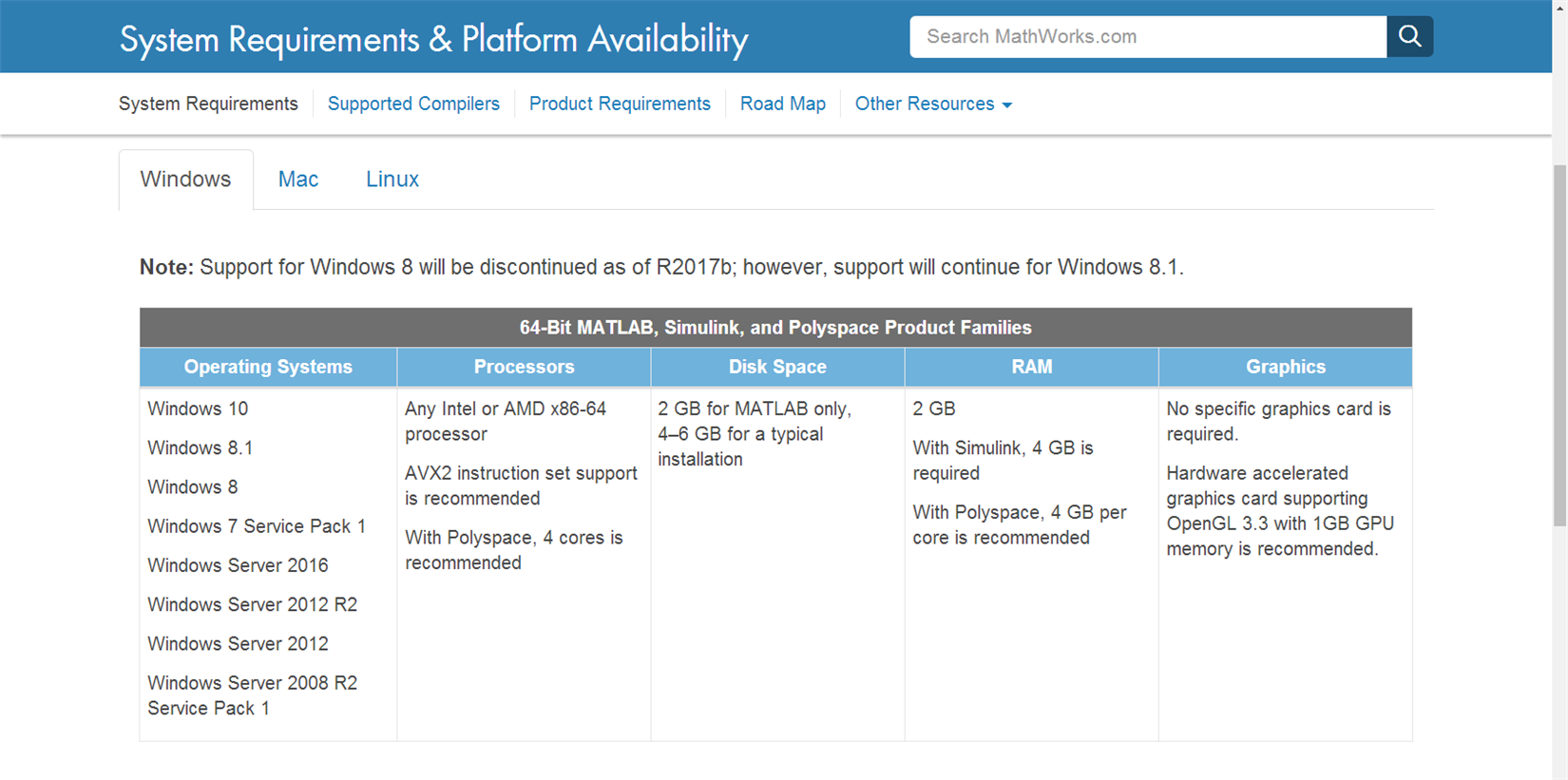•#### MATLAB for Machine Learning#### Overview of this book

MATLAB is the language of choice for many researchers and mathematics experts for machine learning. This book will help you build a foundation in machine learning using MATLAB for beginners. You’ll start by getting your system ready with t he MATLAB environment for machine learning and you’ll see how to easily interact with the Matlab workspace. We’ll then move on to data cleansing, mining and analyzing various data types in machine learning and you’ll see how to display data values on a plot. Next, you’ll get to know about the different types of regression techniques and how to apply them to your data using the MATLAB functions. You’ll understand the basic concepts of neural networks and perform data fitting, pattern recognition, and clustering analysis. Finally, you’ll explore feature selection and extraction techniques for dimensionality reduction for performance improvement. At the end of the book, you will learn to put it all together into real-world cases covering major machine learning algorithms and be comfortable in performing machine learning with MATLAB.
Title PageCreditsForewordwww.PacktPub.comCustomer FeedbackPrefaceFree Chapter
Getting Started with MATLAB Machine LearningImporting and Organizing Data in MATLABFrom Data to Knowledge DiscoveryFinding Relationships between Variables - Regression TechniquesPattern Recognition through Classification AlgorithmsIdentifying Groups of Data Using Clustering MethodsSimulation of Human Thinking - Artificial Neural NetworksImproving the Performance of the Machine Learning Model - Dimensionality ReductionMachine Learning in Practice## Introducing machine learning with MATLAB

So far, we have learned what machine learning algorithms do; we have also understood how to recognize the different types, how to locate the right solution for our needs, and finally how to set a proper workflow. It's time to learn how to do all this in the MATLAB environment.

Solving machine learning problems becomes extremely easy with the use of the tools available in the MATLAB environment. This is because MATLAB is a strong environment for interactive exploration. It has numerous algorithms and apps to help you get started using machine learning techniques. Some examples include:

• Clustering, classification, and regression algorithms
• Neural network app, curve fitting app, and Classification Learner app

MATLAB is a software platform optimized for solving scientific problems and design. In MATLAB, calculation, visualization, and programming are integrated in an easy-to-use environment, where problems and solutions are expressed in familiar mathematical notation.

The name MATLAB is an acronym of the term matrix laboratory. MATLAB was originally written to provide easy access to software of matrices; then it evolved in the years to come, thanks to numerous user inputs. The MATLAB programming language is based on matrices that represent the most natural way to express computational mathematics. Its desktop environment invites experimentation, exploration, and discovery. The integrated graphics are easy to view and provide an in-depth understanding of the data.

The MATLAB desktop is shown in the following screenshot:Figure 1.11: MATLAB desktop

MATLAB is also characterized by the presence of specific solutions to application problems called toolboxes. Very useful for most users, MATLAB toolboxes represent solutions for many practical problems and provide the basis for applying these instruments to the specialized technology. These toolboxes are collections of MATLAB functions (referred to as M-files) that extend the MATLAB environment in order to solve particular classes of problems.

MATLAB has two specific toolboxes for processing machine learning problems. They are the Statistics and Machine Learning Toolbox and Neural Network Toolbox. While the first solves machine learning problems through statistical techniques and algorithms most widely used in this field, the second is specific to ANNs. In the following sections, we will analyze in detail the features of these tools.Figure 1.12: Some apps available in MATLAB

### System requirements and platform availability

To be used efficiently, all computer software needs certain hardware components or other software resources to be present on a computer. Thus, MATLAB requires specific hardware to be installed and working properly on our computer. Fortunately, we can start from the assumption that MATLAB is available for all popular software platforms in both professional and student editions. In fact, it is available for the Windows, macOS, and Linux platforms. But we can rest assured that what MATLAB requires is widely supported by new computers.

So the hardware requirements for Windows are:

• Operating systems: Windows 10, Windows 8.1, Windows 8, Windows 7 Service Pack 1, Windows Server 2016, Windows Server 2012 R2, Windows Server 2012, and Windows Server 2008 R2 Service Pack 1.
• Processors: Any Intel or AMD x86-64 processor; AVX2 instruction set support is recommended; with Polyspace, 4-core is recommended.
• Disk Space: 2 GB for MATLAB only, 4–6 GB for a typical installation.
• RAM: 2 GB; with Simulink, 4 GB is required; with Polyspace, 4 GB per core is recommended.
• Graphics: No specific graphics card is required. A hardware-accelerated graphics card supporting OpenGL 3.3 with 1 GB GPU memory is recommended.

### Note

To discover the hardware requirements for other platforms, visit the manufacturer's website at the following link: https://www.mathworks.com/support/sysreq.html.

In the following screenshot, the hardware requirements for Windows are listed:Figure 1.13: Windows hardware requirements for MATLAB.